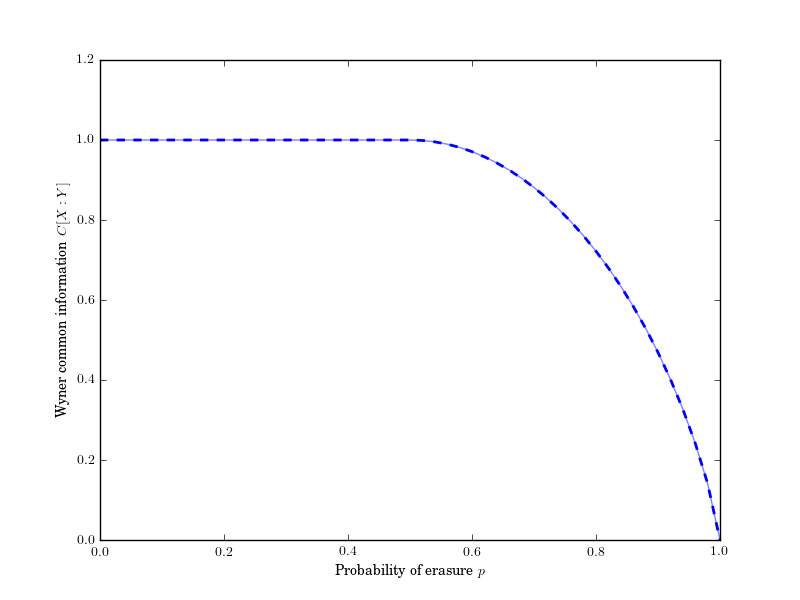# Wyner Common Information¶

The Wyner common information [Wyn75][LXC10] measures the minimum amount of information necessary needed to reconstruct a joint distribution from each marginal.

$\C{X_{0:n} | Y_{0:m}} = \min_{\ind X_{0:n} \mid Y_{0:m}, V} \I{X_{0:n} : V | Y_{0:m}}$

## Binary Symmetric Erasure Channel¶

The Wyner common information of the binary symmetric erasure channel is known to be:

$\begin{split}\C{X : Y} = \begin{cases} 1 & p < \frac{1}{2} \\ \H{p} & p \ge \frac{1}{2} \end{cases}.\end{split}$

We can verify this:

In : In : from dit.multivariate import wyner_common_information as C## API¶

wyner_common_information(dist, rvs=None, crvs=None, niter=None, maxiter=1000, polish=1e-06, bound=None, rv_mode=None)

Computes the wyner common information, min I[X:V] such that V renders all X_i independent.

Parameters
• dist (Distribution) – The distribution for which the wyner common information will be computed.

• rvs (list, None) – A list of lists. Each inner list specifies the indexes of the random variables used to calculate the wyner common information. If None, then it calculated over all random variables, which is equivalent to passing rvs=dist.rvs.

• crvs (list, None) – A single list of indexes specifying the random variables to condition on. If None, then no variables are conditioned on.

• niter (int > 0) – Number of basin hoppings to perform during the optimization.

• maxiter (int > 0) – The number of iterations of the optimization subroutine to perform.

• polish (False, float) – Whether to polish the result or not. If a float, this will perform a second optimization seeded with the result of the first, but with smaller tolerances and probabilities below polish set to 0. If False, don’t polish.

• bound (int) – Bound the size of the Markov variable.

• rv_mode (str, None) – Specifies how to interpret rvs and crvs. Valid options are: {‘indices’, ‘names’}. If equal to ‘indices’, then the elements of crvs and rvs are interpreted as random variable indices. If equal to ‘names’, the the elements are interpreted as random variable names. If None, then the value of dist._rv_mode is consulted, which defaults to ‘indices’.

Returns

ci – The wyner common information.

Return type

float